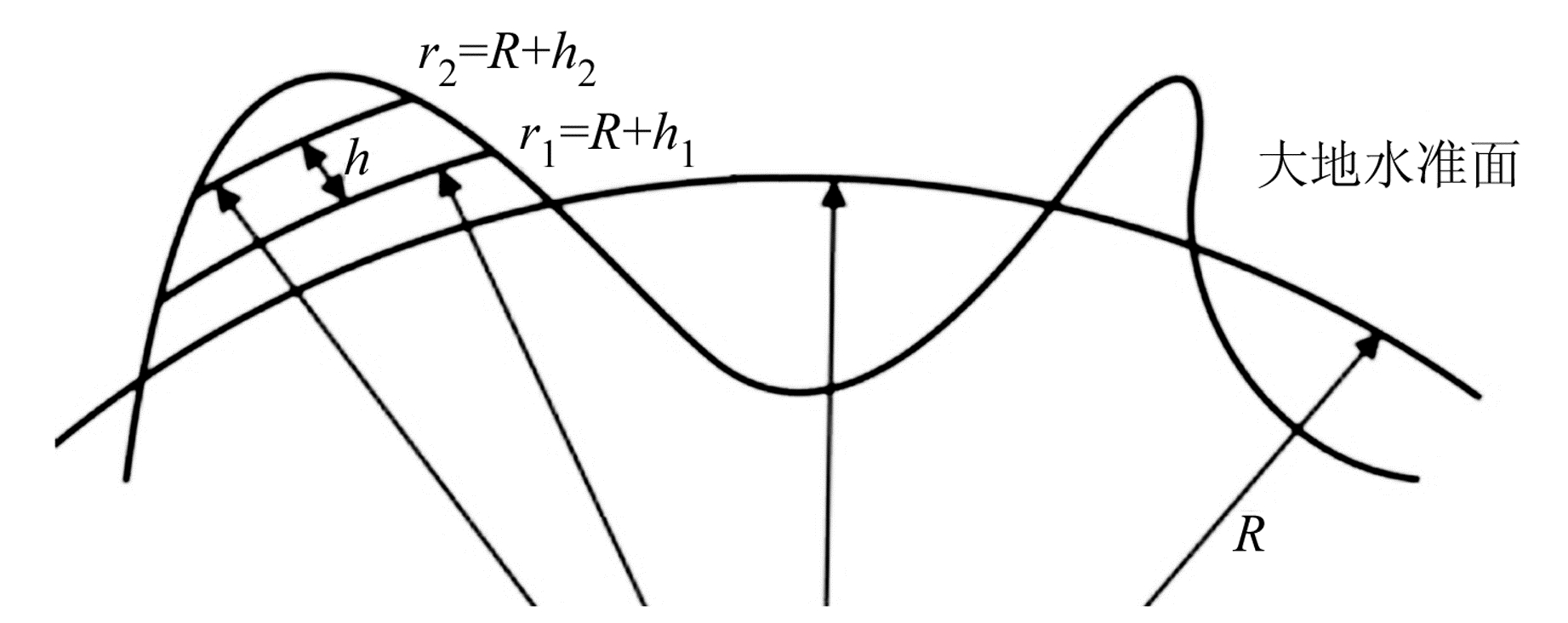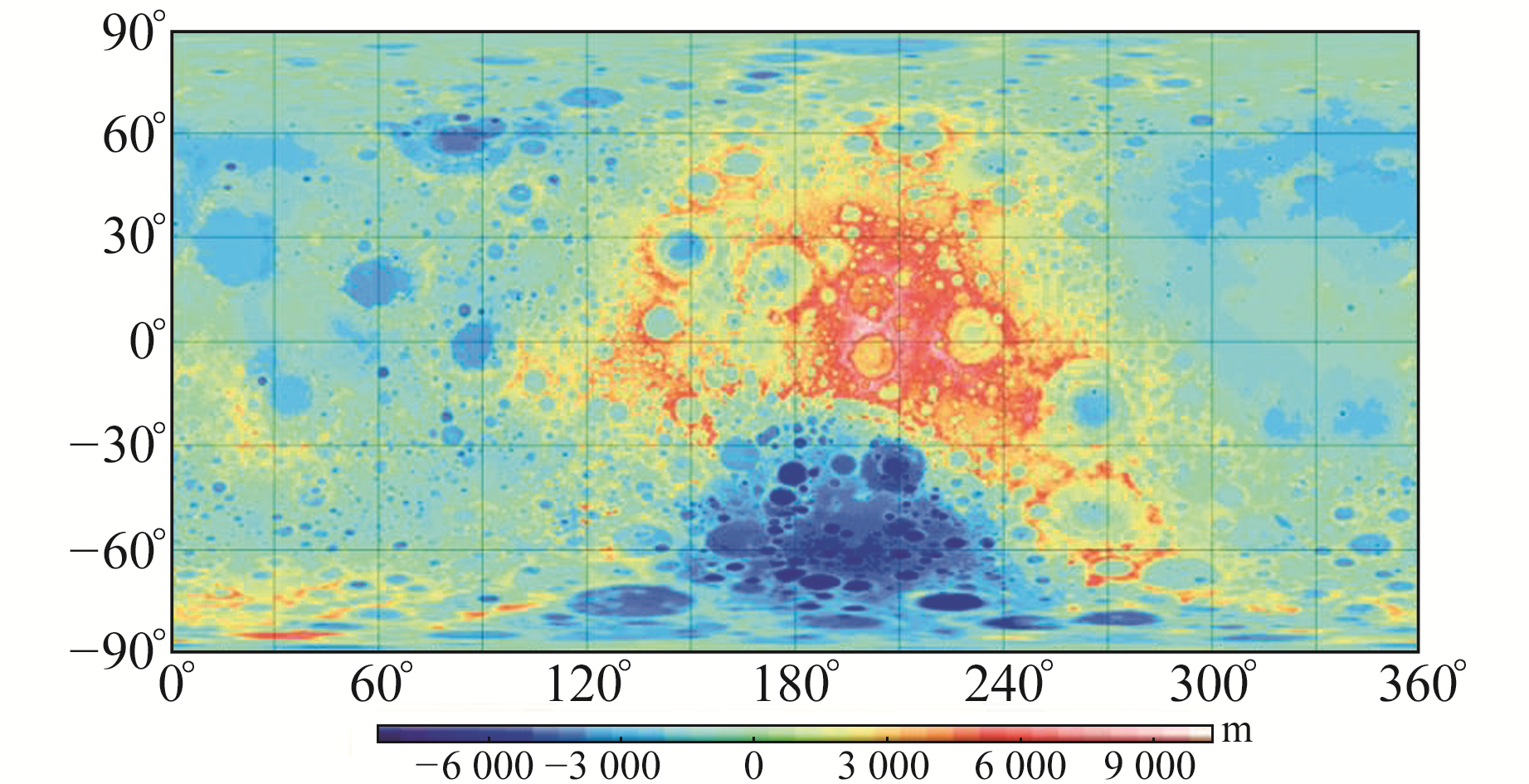﻿ 利用全张量重力梯度异常探测月球地下隐伏地质结构及其解释文章快速检索 高级检索
 大地测量与地球动力学2023, Vol. 43Issue (4): 409-413, 440  DOI: 10.14075/j.jgg.2023.04.014### 引用本文YE Zhourun, LIANG Xinghui, LIU Jinzhao, et al. Exploration and Interpretation of Lunar Underground Concealed Structures Using Full Gravity Gradients Anomaly[J]. Journal of Geodesy and Geodynamics, 2023, 43(4): 409-413, 440.### Foundation support

National Natural Science Foundation of China, No.41904010;Natural Science Foundation of Anhui Province, No.2008085MD115;Open Fund of State Key Laboratory of Geodesy and Earth's Dynamics, Innovation Academy for Precision Measurement Science and Technology, CAS, No.SKLGED2022-1-4; Fundamental Research Funds for the Central Universities, No.JZ2021HGTB0107.

### Corresponding author

LIANG Xinghui, PhD, associate researcher, majors in physical geodesy, E-mail: lxh_whigg@whigg.ac.cn.

### 第一作者简介

YE Zhourun, PhD, associate professor, majors in physical geodesy, E-mail: yezhorun329@hotmail.com.

### 文章历史

1. 合肥工业大学土木与水利工程学院，合肥市屯溪路193号，230009;
2. 中国科学院精密测量科学与技术创新研究院大地测量与地球动力学国家重点实验室，武汉市徐东大街340号，430077;
3. 中国地震局第一监测中心，天津市耐火路7号，300180

1 研究方法 1.1 月球自由空气扰动重力梯度获取

 $\begin{gathered} T(r, \theta, \lambda)=\frac{G M}{R} \sum\limits_{n=N_{\min }}^{N_{\max }} \sum\limits_{m=0}^n\left(\frac{R}{r}\right)^{n+1} \cdot \\ \left(\bar{C}_{n m} \cos (m \lambda)+\bar{S}_{n m} \sin (m \lambda)\right) \bar{P}_{n m}(\cos \theta) \end{gathered}$ (1)

 \begin{aligned} & T_{x x}=\frac{1}{r^2}\left(T_{\theta \theta}+r T_r\right) \\ & T_{x y}=T_{y x}=\frac{1}{r^2 \sin \theta}\left(T_{\lambda \theta}-\frac{\cos \theta}{\sin \theta} T_\lambda\right) \\ & T_{x z}=T_{z x}=\frac{1}{r}\left(\frac{1}{r} T_\theta-T_{r \theta}\right) \\ & T_{y y}=\frac{1}{r^2 \sin ^2 \theta}\left(T_{\lambda\lambda}+r \sin ^2 \theta T_r+\right. \\ &\left.\cos \theta \sin \theta T_\theta\right) \\ & T_{y z}=T_{z y}=\frac{1}{r \sin \theta}\left(T_{\lambda r}-\frac{1}{r} T_\lambda\right) \\ & T_{z z}=T_{r r} \end{aligned} (2)

1.2 地形高程获取

 $\begin{gathered} H(\theta, \lambda)=\sum\limits_{n=0}^{N_{\max }^{\mathrm{DTM}}} \sum\limits_{m=0}^n\left(\overline{\mathrm{HC}}_{n m} \cos (m \lambda)+\right. \\ \left.\overline{\mathrm{HS}}_{n m} \sin (m \lambda)\right) \bar{P}_{n m}(\cos \theta) \end{gathered}$ (3)

1.3 基于有限功率地形法的地形重力梯度改正

 $C_{m m}^\beta=\left\{\begin{array}{l} \bar{C}_{n m} \\ \bar{S}_{n m} \end{array}\right\}=\frac{3}{2 n+1} \frac{1}{\bar{\rho}}\left(\frac{H_{n m}^1}{R}+\frac{(n+2) H_{n m}^2}{2 R^2}+\right. \\ \quad\quad\quad\quad\quad \left.\frac{(n+2)(n+1) H_{n m}^3}{6 R^3}\right)$ (4)

 $\begin{gathered} H_{n m}^i=\frac{1}{4 \pi} \int_{\lambda_1=0}^{\lambda_2=2 \pi} \int_{\theta_1=0}^{\theta_2=\pi}\left(h_2^i-h_1^i\right) \cdot \\ \rho\left(r^{\prime}, \theta^{\prime}, \lambda^{\prime}\right) Y_{m m}^\beta\left(r^{\prime}, \theta^{\prime}, \lambda^{\prime}\right) \sin \theta^{\prime} \mathrm{d} \theta^{\prime} \mathrm{d} \lambda^{\prime} \end{gathered}$ (5)
 $Y_{n m}^\beta(\theta, \lambda)=\bar{P}_{m m}(\cos \theta)\left\{\begin{array}{l} \cos m \lambda, \beta=0 \\ \sin m \lambda, \beta=1 \end{array}\right.$ (6)图 1 地形起伏示意图 Fig. 1 Schematic view of topography
2 实验计算和讨论表 1 原始扰动重力梯度 Tab. 1 Original disturbing gravity gradient图 2 原始扰动重力梯度 Fig. 2 Original disturbing gravity gradient图 3 月球地形起伏 Fig. 3 Lunar topographic relief表 2 地形校正后重力梯度异常 Tab. 2 Gravity gradient anomaly after terrain correction图 4 地形校正后重力梯度异常 Fig. 4 Gravity gradient anomaly after terrain correction表 3 质量瘤地下分布范围 Tab. 3 Underground range distribution of main mascons

3 结语

  Hirt C, Yang M, Kuhn M, et al. SRTM2 Gravity: An Ultrahigh Resolution Global Model of Gravimetric Terrain Corrections[J]. Geophysical Research Letters, 2019, 46(9): 4 618-4 627 DOI:10.1029/2019GL082521 (0)  Neumann G A, Zuber M T, Smith D E, et al. The Lunar Crust: Global Structure and Signature of Major Basins[J]. Journal of Geophysical Research: Planets, 1996, 101(E7): 16 841-16 863 DOI:10.1029/96JE01246 (0)  Heck B, Seitz K. A Comparison of the Tesseroid, Prism and Point-Mass Approaches for Mass Reductions in Gravity Field Modelling[J]. Journal of Geodesy, 2007, 81(2): 121-136 DOI:10.1007/s00190-006-0094-0 (0)  Wieczorek M A, Phillips R J. Potential Anomalies on A Sphere: Applications to the Thickness of the Lunar Crust[J]. Journal of Geophysical Research: Planets, 1998, 103(E1): 1 715-1 724 DOI:10.1029/97JE03136 (0)  Tsoulis D, Stary B. An Isostatically Compensated Gravity Model Using Spherical Layer Distributions[J]. Journal of Geodesy, 2004, 78(7-8): 418-424 DOI:10.1007/s00190-004-0404-3 (0)  Šprlák M, Han S C. On the Use of Spherical Harmonic Series Inside the Minimum Brillouin Sphere: Theoretical Review and Evaluation by GRAIL and LOLA Satellite Data[J]. Earth-Science Reviews, 2021, 222 (0)  Heiskanen W A, Moritz H. Physical Geodesy[M]. Beijing: Surveing and Mapping Press, 1979 (0)  Zhu L Z, Jekeli C. Gravity Gradient Modeling Using Gravity and DEM[J]. Journal of Geodesy, 2009, 83(6): 557-567 DOI:10.1007/s00190-008-0273-2 (0)  Pavlis N K, Holmes S A, Kenyon S C, et al. The Development and Evaluation of the Earth Gravitational Model 2008(EGM2008)[J]. Journal of Geophysical Research: Solid Earth, 2012, 117(B4) (0)  Wirnsberger H, Krauss S, Mayer-Gürr T. First Independent Graz Lunar Gravity Model Derived from GRAIL[J]. Icarus, 2019, 317: 324-336 DOI:10.1016/j.icarus.2018.08.011 (0)  Bucha B, Hirt C, Kuhn M. Divergence-Free Spherical Harmonic Gravity Field Modeling Based on the Runge-Krarup Theorem: A Case Study for the Moon[J]. Journal of Geodesy, 2019, 93(4): 489-513 DOI:10.1007/s00190-018-1177-4 (0)  Liang Q, Chen C, Li Y G. 3-D Inversion of Gravity Data in Spherical Coordinates with Application to the GRAIL Data[J]. Journal of Geophysical Research: Planets, 2014, 119(6): 1 359-1 373 DOI:10.1002/2014JE004626 (0)  Zuber M T, Smith D E, Watkins M M, et al. Gravity Field of the Moon from the Gravity Recovery and Interior Laboratory(GRAIL) Mission[J]. Science, 2013, 339(6 120): 668-671 (0)  Neumann G A, Zuber M T, Wieczorek M A, et al. Lunar Impact Basins Revealed by Gravity Recovery and Interior Laboratory Measurements[J]. Science Advances, 2015, 1(9) (0)  Andrews-Hanna J C, Asmar S W, Head J W, et al. Ancient Igneous Intrusions and Early Expansion of the Moon Revealed by GRAIL Gravity Gradiometry[J]. Science, 2013, 339(6 120): 675-678 (0)  陆天启, 陈圣波, 朱凯. 基于GRAIL重力数据的月球深部断裂识别和空间分布研究[J]. 地球物理学报, 2019, 62(8): 2 835-2 844 (Lu Tianqi, Chen Shengbo, Zhu Kai. Global Identification and Spatial Distribution of Lunar Subsurface Faults from GRAIL Gravity Data[J]. Chinese Journal of Geophysics, 2019, 62(8): 2 835-2 844) (0)  Saad A H. Understanding Gravity Gradients-A Tutorial[J]. The Leading Edge, 2006, 25(8): 942-949 DOI:10.1190/1.2335167 (0)  Zhao G D, Chen B, Uieda L, et al. Efficient 3-D Large-Scale forward Modeling and Inversion of Gravitational Fields in Spherical Coordinates with Application to Lunar Mascons[J]. Journal of Geophysical Research: Solid Earth, 2019, 124(4): 4 157-4 173 DOI:10.1029/2019JB017691 (0)  马宗晋, 高祥林, 宋正范. 中国布格重力异常水平梯度图的判读和构造解释[J]. 地球物理学报, 2006, 49(1): 106-114 (Ma Zongjin, Gao Xianglin, Song Zhengfan. Analysis and Tectonic Interpretation to the Horizontal-Gradient Map Calculated from Bouguer Gravity Data in the China Mainland[J]. Chinese Journal of Geophysics, 2006, 49(1): 106-114) (0)  Melosh H J. Global Tectonics of a Despun Planet[J]. Icarus, 1977, 31(2): 221-243 DOI:10.1016/0019-1035(77)90035-5 (0)
Exploration and Interpretation of Lunar Underground Concealed Structures Using Full Gravity Gradients Anomaly
YE Zhourun1,2LIANG Xinghui2LIU Jinzhao3     LIU Lintao2
1. College of Civil Engineering, Hefei University of Technology, 193 Tunxi Road, Hefei 230009, China;
2. State Key Laboratory of Geodesy and Earth's Dynamics, Innovation Academy for Precision Measurement Science and Technology, CAS, 340 Xudong Street, Wuhan 430077, China;
3. The First Monitoring and Application Center, CEA, 7 Naihuo Road, Tianjin 300180, China
Abstract: We apply the finite power terrain correction method(i.e., the Tesseroid forward modelling in harmonic spectral domain) to the gravity gradient calculation in terms of lunar topography. Using gravity data from the Gravity Recovery and Interior Laboratory(GRAIL) mission and terrain information recovered from the Moontopo720 model, we calculate the total gravity tensors anomaly after terrain correction at the height of GRAIL satellite. By taking advantage of the multi-component characteristics of gravity gradient and the relevant experimental results, we estimate the spatial horizontal range distributions of underground mascons through the horizontal gradient anomaly. Using the total gravity gradient anomaly maps, we preliminarily verify the distributions of main fault structures caused by tidal gravity.
Key words: Moon; gravity gradient; terrain correction; Tesseroid model